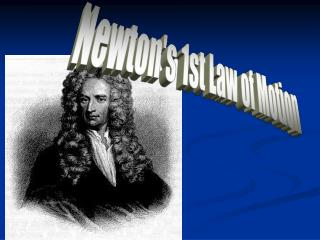DownloadDownload PresentationNewton's 1st Law of Motion

# Newton's 1st Law of Motion

Download Presentation## Newton's 1st Law of Motion

- - - - - - - - - - - - - - - - - - - - - - - - - - - E N D - - - - - - - - - - - - - - - - - - - - - - - - - - -
##### Presentation Transcript

1. Newton's 1st Law of Motion

2. Forces • A force is a push or a pull • any influence that can change the velocity of an object • There can be no acceleration without a force • is a vector having both magnitude and direction. Like any other vectors, force vectors are represented by arrows.

3. Newton’s Laws of Motion • An object at rest stays at rest until an outside force causes it to move. • An object in motion continues to move in the same direction at the same speed until a force stops it or changes its direction. • So, an object at rest will stay at rest, and an object in motion will remain in motion unless acted by an outside force. • Newton’s First Law of Motion is also known as the Law of Inertia

4. Inertia • Inertia is an objects resistance to a change in motion. • The inertias between two objects can be compared when a spring is used to accelerate different masses • The acceleration of an object depends on how much force is applied to an object's mass.

5. Inertia Example An astronaut in outer space will continue drifting in the same direction at the same speed indefinitely, until acted upon by an outside force.

6. Mass • The quantitative measure of the inertia of an object is an object's mass • Mass refers to the quantity of matter in an object. If it balances, the masses are equal. Einstein asserted that these two kinds of masses are equivalent. ? m Balance

7. Weight • Weight is the pull of gravity exerted on an object (with mass). • weight = mass x acceleration of gravity • Near the surface of the Earth, g = 10 m/s2. • Mass is recorded in Kilograms, while weight is recorded in Newtons. • Newton = Kg x m/s2

8. Force Diagrams • By drawing all the force vectors acting around an object you can create a force diagram. • Free-body diagrams are a very important part of determining how forces are acting on an object. N f= 22 N 38 N 8 kg mg

9. Net Force • The Net Force is the combination of all forces acting on an object and can be determined through vector addition. • Unbalanced forces make objects accelerate. • Balanced forces are at equilibrium (no acceleration) and have a Net Force of Zero • Like a tug of war F1 F2 F3 Fnet

10. Balanced Forces

11. Unbalanced Forces • The forces don’t cancel out • Cause a change in motion • Act as one force

12. Friction • Friction always opposes the motion of an object. • The friction forces are parallel to the contact surface and occur when… • One body slides over the other, or… • They cling together despite and external force. • The forces shown are an action-reaction pair. v f Acme Hand Grenades

13. Two Kinds of Friction FA fs • Static friction • Must be overcome in order to budge an object • Present only when there is no relative motion between the bodies, e.g., the box & table top • Kinetic friction • Weaker than static friction • Present only when objects are moving with respect to each other (skidding) objects still or moving together FA fk a to the right v left or right

14. Tension Force • The force of Tension exerted on a cable is equal in magnitude to the force of Weight in the object being held by the cable, but in the opposite direction, if the object is in Equilibrium. T m mg

15. Normal force • When an object lies on a table or on the ground, the table or ground must exert an upward force on it, otherwise gravity would accelerate it down. • This force is called the normal force. N In this particular case, N = mg. So, Fnet = 0; hence a = 0. m mg

16. Normal forces aren’t always up “Normal” means perpendicular. A normal force is always perpendicular to the contact surface. N For example, if a flower pot is setting on an incline, N is not vertical; it’s at a right angle to the incline. Also, in this case, mg > N. mg

17. Air Resistance • The force of the air against a moving object • Increases as the velocity of the motion increases • The size and shape of the object also effect the air resistance • Larger surface area more resistance • Car designers try to minimize it • Overcoming air resistance uses more fuel

18. Terminal velocity • Force of gravity is constant • air resistance increases as you speed up • until the force is equal • Equal forces, no acceleration • constant velocity terminal velocity